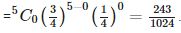Courses

# Test: Probability- 2

## 25 Questions MCQ Test Mathematics (Maths) Class 12 | Test: Probability- 2

Description
This mock test of Test: Probability- 2 for JEE helps you for every JEE entrance exam. This contains 25 Multiple Choice Questions for JEE Test: Probability- 2 (mcq) to study with solutions a complete question bank. The solved questions answers in this Test: Probability- 2 quiz give you a good mix of easy questions and tough questions. JEE students definitely take this Test: Probability- 2 exercise for a better result in the exam. You can find other Test: Probability- 2 extra questions, long questions & short questions for JEE on EduRev as well by searching above.
QUESTION: 1

### Two coins are tossed once ,where E : tail appears on one coin , F : one coin shows head. Find P(E/F).

Solution: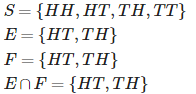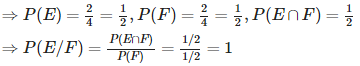QUESTION: 2

### An instructor has a question bank consisting of 300 easy True / False questions,200 difficult True / False questions, 500 easy multiple choice questions and 400 difficult multiple choice questions. If a question is selected at random from the question bank, what is the probability that it will be an easy question given that it is a multiple choice question?

Solution: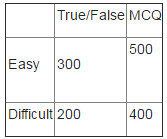Total = 1400, therefore n(S) = 1400 Let A = event of getting easy question B = event of getting multiple choice question.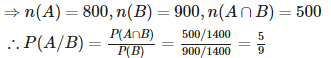QUESTION: 3

### Let A and B be independent events with P (A) = 0.3 and P(B) = 0.4.Find P(A ∪ B)

Solution:

Let A and B be independent events with P (A) = 0.3 and P(B) = 0.4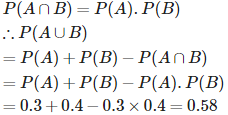QUESTION: 4

Let X be a random variable assuming values x1, x2,....,xn with probabilities p1, p2, ...,pn, respectively such that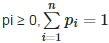. If E is the expectation, mean of X is denoted by μ, variance denoted by σ2, is defined as

Solution:

Let X be a random variable assuming values x1, x2,....,xn with probabilities p1, p2, ...,pn, respectively such that. If E is the expectation, mean of X is denoted by μμ, variance denoted by σ2, is defined as : σ2=E(X – μ)2

QUESTION: 5

A die is thrown 6 times. If ‘getting an odd number’ is a success, what is the probability of at most 5 successes?

Solution:

p = probability of success = 3/6 = ½ . q = probability of failure = 1 – p = 1 – ½ = ½ . let x be the number of successes , then x has the binomial distribution with : n = 6 , p = ½ , q = ½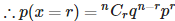P(atmost 5 successes ) = P ( x ≤ 5 ) = 1 - P(x > 5) = 1 – P(x = 6)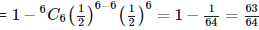QUESTION: 6

Two coins are tossed once ,where E :no tail appears , F : no head appers. Find P(E/F).

Solution: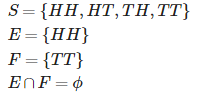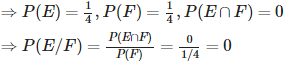QUESTION: 7

Consider the experiment of throwing a die, if a multiple of 3 comes up, throw the die again and if any other number comes, toss a coin. Find the conditional probability of the event ‘the coin shows a tail’, given that ‘at least one die shows a 3’.

Solution:

S = {(3,1), (3,2), (3,3), (3,4), (3,5), (3,6), (6,1), (6,2), (6,3), (6,4), (6,5), (6,6), (1,H), (2,H), (4,H), (5,H), (1,T), (2,T), (4,T), (5,T)} Let A = event that coin shows a tail. i.e. A = {(1,T), (2,T), (4,T), (5,T)} and B = event that atleast one die shows 3. B = {(3,1), (3,2), (3,3), (3,4), (3,5), (3,6),(6,3)}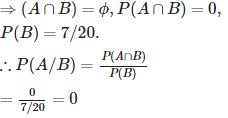QUESTION: 8

Let A and B be independent events with P (A) = 0.3 and P(B) = 0.4. Find P (A|B)

Solution:

Let A and B be independent events with P (A) = 0.3 and P(B) = 0.4 P(A/B)=P(A)=0.3.

QUESTION: 9

A random variable X taking values 0, 1, 2, ..., n is said to have a binomial distribution with parameters n and p, if its probability distribution is given by

Solution:

A random variable X taking values 0, 1, 2, ..., n is said to have a binomial distribution with parameters n and p, if its probability distribution is given by :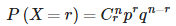QUESTION: 10

A pair of dice is thrown 4 times. If getting a doublet is considered a success, find the probability of two successes.

Solution:

When a die is tossed , then the doublets are {(1,1),(2,2),(3,3),(4,4),(5,5),(6,6)}. Therefore, p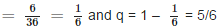. Let x be the number of successes , then x has the binomial distribution with : n = 4 , p = 1/6 , q = 5/6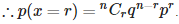P (2 successes) = P (x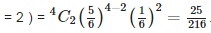QUESTION: 11

Given that E and F are events such that P(E) = 0.6, P(F) = 0.3 and P(E ∩ F) = 0.2, find P (E|F) and P(F|E)

Solution:

We have ,
P(E) = 0.6, P(F) = 0.3 and P(E ∩ F) = 0.2, then ,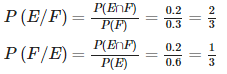QUESTION: 12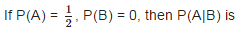Solution: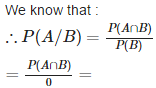QUESTION: 13

Let A and B be independent events with P (A) = 0.3 and P(B) = 0.4. Find P(B|A).

Solution:

Let A and B be independent events with P (A) = 0.3 and P(B) = 0.4. P(B/A)=P(B)=0.4

QUESTION: 14

State which of the following is a probability distribution of a random variable.

Solution: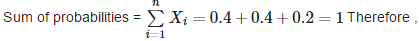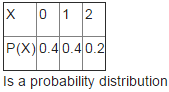QUESTION: 15

Five cards are drawn successively with replacement from a well – shuffled deck of 52 cards. What is the probability that all the five cards are spades?

Solution:

Here , probability of getting a spade from a deck of 52 cards = 13/52 = ¼ . p = ¼ , q = ¾ . let , x is the number of spades , then x has the binomial distribution with n = 5 , p = ¼ , q = ¾ . P( all 5 cards are spades)= P (x = 5) =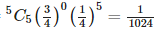QUESTION: 16

Compute P(A|B), if P(B) = 0.5 and P (A ∩ B) = 0.32

Solution: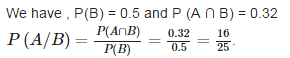QUESTION: 17

If A and B are events such that P(A|B) = P(B|A), then

Solution: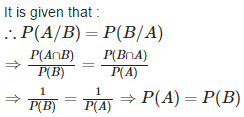QUESTION: 18

If A and B are two events such that P(A) = ¼ , P(B) = ½ and P(A∩B) =1/8 , Find P(not A and not B) .

Solution:

Since A and B are independent events .not A and not B are also independent events .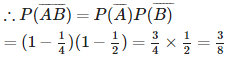QUESTION: 19

An urn contains 5 red and 2 black balls. Two balls are randomly drawn. Let X represent the number of black balls. What are the possible values of X? Is X a random variable ?

Solution:

An urn contains 5 red and 2 black balls. Two balls are randomly drawn. Let X represent the number of black balls.therefore the possible values of X = 0 , 1 ,2 . Yes , X is a random variable.

QUESTION: 20

Five cards are drawn successively with replacement from a well – shuffled deck of 52 cards. What is the probability that only 3 cards are spades?

Solution:

Here , probability of getting a spade from a deck of 52 cards = 13/52 = ¼ . p = ¼ , q = ¾ . let , x is the number of spades , then x has the binomial distribution with n = 5 , p = ¼ , q = ¾ . P( only 3 cards are spades) = P (x = 3)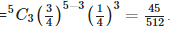QUESTION: 21

If P (A) = 0.8, P (B) = 0.5 and P(B|A) = 0.4, find P(A ∩ B)

Solution: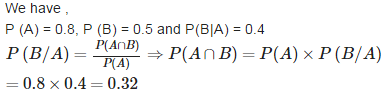QUESTION: 22

If E and F are independent, then

Solution:

If E and F are independent, then P (E ∩ F) = P (E) P (F).

QUESTION: 23

If A and B are two events such that A ⊂ B and P(B) ≠ 0, then which of the following is correct?

Solution:

If A and B are two events such that A ⊂ B and P(B) ≠ 0, then , P(A|B) ≥ P(A).

QUESTION: 24

Let X represent the difference between the number of heads and the number of tails obtained when a coin is tossed 6 times. What are possible values of X?

Solution:

Let X represent the difference between the number of heads and the number of tails obtained when a coin is tossed 6 times. When a coin is tossed 6 times , we have 64 outcomes which consists of : (i) 6 heads and 0 tails (ii) 5 heads and 1 tail (iii) 4 heads and 2 tails (iv) 3 heads and 3 tails (v) 2 heads and 4 tails (vi) 1 head and 5 tails (vii) 0 head and 6 tails . Let X represents the difference between the number of heads and tails. (i) ⇒ X = 6 – 0 = 6 (ii) ⇒ X = 5 – 1 = 4 (iii) ⇒ X = 4 – 2 = 2 (iv) ⇒ X = 3 – 3 = 0 (v) ⇒ X = 4 – 2 = 2 (vi) ⇒ X = 5 – 1 = 4 (vii) ⇒ X = 6 – 0 = 6 . Therefore , X = 6 , 4 , 2, 0 .

QUESTION: 25

Five cards are drawn successively with replacement from a well – shuffled deck of 52 cards. What is the probability that none is a spade?

Solution:

Here , probability of getting a spade from a deck of 52 cards = 13/52 = ¼ . p = ¼ , q = ¾ .
let , x is the number of spades ,
then x has the binomial distribution with n = 5 , p = ¼ , q = ¾ .
P( none is a spade)= P (x = 0)